Orginal Article

# 密集颗粒体系的颗粒运动及结构测量技术

1上海理工大学光电信息与计算机工程学院, 上海 200093
2 北京科技大学物理系, 北京 100083
3 上海交通大学物理与天文学院, 上海 200240
4 清华大学水沙科学与水利水电工程国家重点实验室, 北京 100084

# Measurement techniques of grain motion and inter-grain structures in dense granular materials

YANG Hui1, ZHANG Guohua2, WANG Yujie3, SUN Qicheng4ɛ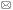1 School of Optical-Electrical and Computer Engineering, University of Shanghai for Science and Technology, Shanghai 200093, China
2 Department of Physics, University of Science and Technology Beijing, Beijing 100083, China
3 Department of Physics and Astronomy, Shanghai Jiao Tong University, Shanghai 200240, China
4 State Key Laboratory of Hydroscience and Engineering, Tsinghua University, Beijing 100084, China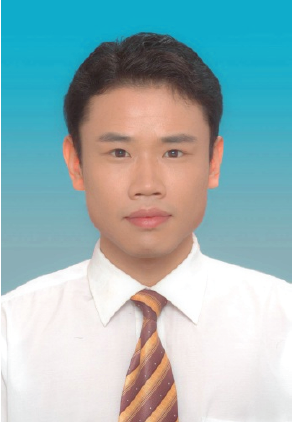Abstract

A dense granular material is composed of a large number of densely packed coarse grains, which is widely encountered in nature, engineering and industries, such as granular debris flows, rock-filled dams, and pebble beds in nuclear reactors. It is a typical many-body system with intrinsic characteristics of structural and dynamic heterogeneities which leads to the complicated macroscopic behavior. By developing the statistic mechanics of structure and dynamics of grains, continuum theories of granular materials can be established and such macroscopic behaviors are then expected to be described. However, it is very difficult to fulfil these objectives because of the limitations of present measurement techniques and theoretical basis. The present measurement techniques are only applicable to study ideal and simple structure problems, and do not work well for granular structures. The shortage of granular structures properties makes the connections between structure and macroscopic properties impossible. Therefore, measurements of heterogeneity in structural and dynamics are the foundation for understanding the complex bulk properties of granular materials. The authors of this paper, from different research institutions, have conducted thorough experimental studies in the past decade, which can be classified into two categories: (1) non-invasive methods, mainly including digital image velocimetry, speckle visibility spectroscopy and Xray-CT, are developed to measure the moment of single grains; (2)response spectrum methods, mainly including volume response spectrum, mechanic response spectrum and acoustic measurements, are developed to directly or indirectly detect the inter-particle contact force. In this paper, we review the fundamental principles of these methods and their limitations, and state-of-the-art studies. Finally, we conclude the paper with our experience sand lessons learned, and provide suggestions for the future work.

Keywords： granular materials ; mesoscopic structure ; structural heterogeneity ; dynamics heterogeneity ; non-invasive method ; response spectrum method ; acoustic spectrum analysis

0

YANG Hui, ZHANG Guohua, WANG Yujie, SUN Qicheng. Measurement techniques of grain motion and inter-grain structures in dense granular materials[J]. 力学进展, 2018, 48(0): 1812- https://doi.org/10.6052/1000-0992-17-010

## 2 数字图像测速法

### 2.1 粒子图像测速技术(PIV)和粒子跟踪测速技术(PTV)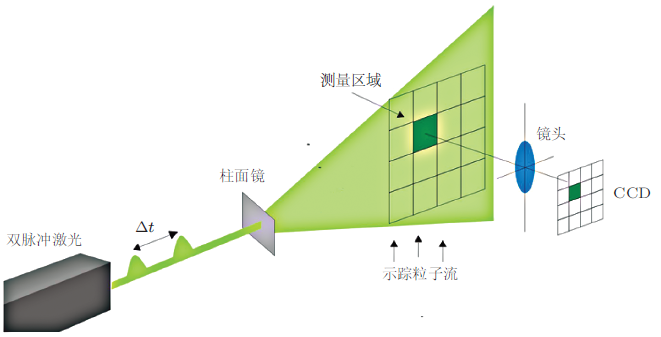PIV技术测量三维空间体内完整速度场是近年来的研究热点.研究曾尝试采用离焦成像、多视角成像和全息成像等方法通过对示踪粒子进行三维空间定位, 实现粒子追踪测速. 受粒子浓度的限制, 空间分辨率并不理想, 直到层析PIV概念的提出, 才使得三维PIV测量技术真正进入了实用阶段(Gao et al. 2013), 该技术通过三维互相关分析来得到具有较高空间分辨率的速度场, 实现了在较高粒子浓度下的测量. 三维PIV的核心技术包括粒子重构和速度场再现技术, 前者采用了医学领域光学层析技术中基于投影方法的代数重构技术, 后者采用三维互相关分析算法(Gao et al. 2011, Percin et al. 2011, Tang et al. 2012).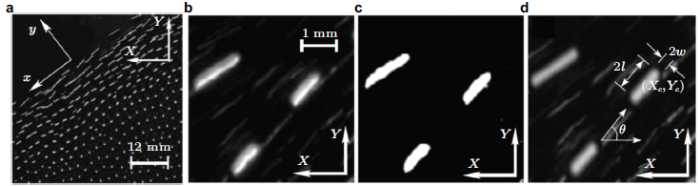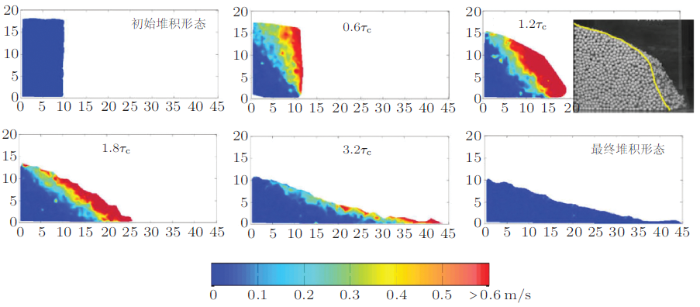PIV和PTV技术的时空分辨率主要由高速相机决定, 其最大的优势在于不仅能追踪到单个颗粒的运动, 而且可以获得全场颗粒速度和加速度, 是目前应用最广泛的一种速度场测量方法.在测量密集颗粒体系时, 其局限性主要是图像中的待测颗粒必须能被有效识别, 才能进行后续计算, 因此适合测量颗粒流表面或二维颗粒流的速度场, 且不能测量小颗粒或不规则颗粒等图像无法有效识别的颗粒体系.

### 2.2 空间滤波测速技术(SFV)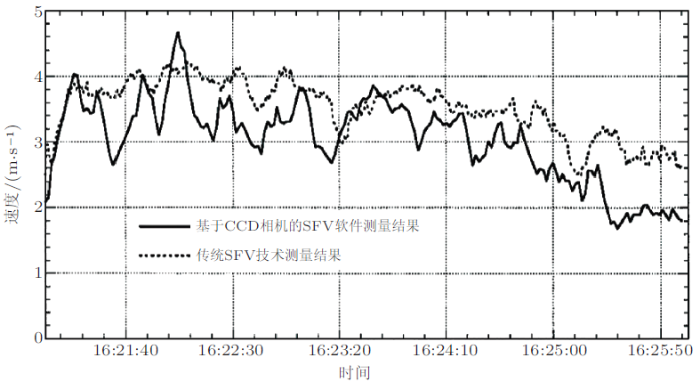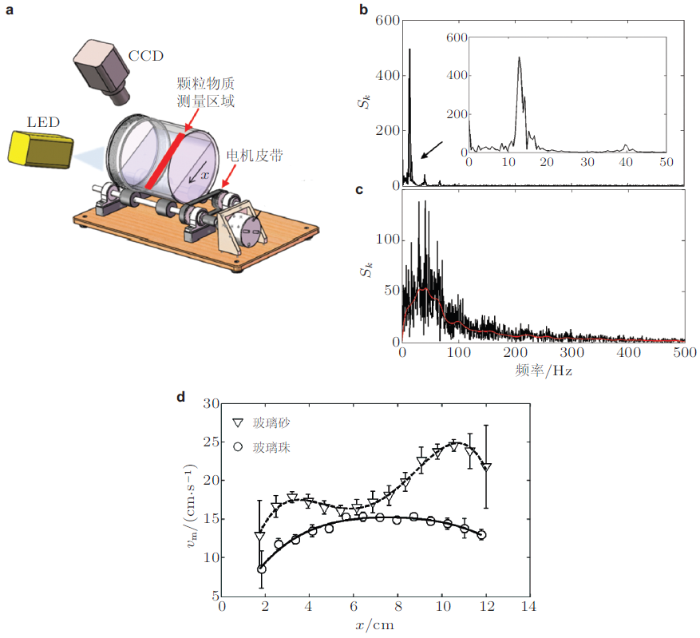## 3 散斑能见度光谱法测量颗粒温度

1905年, 爱因斯坦认为颗粒无序运动与微观粒子热运动有相似性; 直至1978年, Ogawa首次提出了颗粒温度 (granular temperature, $$T_{g})$$概念表征颗粒运动速度的无序程度, $$T_g \equiv\dfrac{1}{3}\left\langle {\left( {\delta v} \right)^2}\right\rangle$$, 其中$$\delta v = v - \left\langle v \right\rangle$$ 是颗粒脉动速度, $$\left\langle { \cdots} \right\rangle$$表示系综平均. 40多年以来, 以颗粒温度为核心概念, 建立了适用于稀疏颗粒流的颗粒动理学理论 (granular kinetics), 得到了稀疏颗粒流的黏滞系数和能量耗散等的解析表达, 但是颗粒温度的实验精细测量还缺乏. 散斑能见度光谱法(speckle visibility spectroscopy, SVS)测量颗粒温度是通过计算一定曝光时间内单幅散斑图像的对比度得到散斑的变化快慢, 进而得到颗粒流的速度脉动信息 (Dixon & Durian 2002, Bandyopadhyay et al. 2005). 由于散斑图像是基于散射光场干涉的原理, 因此其空间分辨率可达到0.1 $$\mu$$m. 另一方面, 由于其采用线阵CCD相机测量散斑对比度变化, 因此其时间分辨率可达到1 $$\mu$$s.

SVS技术是在扩散波光谱(diffusing wave spectroscopy, DWS)技术上发展起来的(Pine et al. 1988), 其原理如图8(a)所示, 激光经过凹透镜扩束后照射在密集的颗粒样品表面, 一部分光子在颗粒间多次散射后从入射反方向离开, 在CCD相机感光面上形成散斑. 当散射区域内颗粒发生运动时, 光子的光程就会发生变化, 从而引起散斑图像产生变化, 称为"动态散斑"或"时变散斑", 并且散斑的变化速率与颗粒的脉动速度有关. 图8(b)显示了不同脉动速度下线阵CCD相机检测的颗粒流散斑图像随时间变化, 可以看到在一定曝光时间下, 散斑图像的对比度随颗粒脉动速度的增大而降低. 根据统计光学理论, 散斑图像的对比度$V_2 (T) = \left( {\dfrac{\sigma _T }{\left\langle I \right\rangle_T }} \right)^2 = \dfrac{\left\langle {I^2} \right\rangle _T}{\left\langle I \right\rangle _T^2 } - 1 \ \ (7)$ 其中, $$I$$为散射光强, $$T$$为曝光时间, $$\left\langle I \right\rangle _T$$和$$\sigma _T ^2$$ 分别为光强的均值和方差.结合高斯光束的Siegert公式, 由式(7)可以得到$V_2 (T) = \beta \int_0^T {2(1 - t/T)} [g_1 (t)]^2{{\rm d}t}/T \ \ (8)$ 其中, $$\beta$$ 为光学系统的相干因子, $$g_1 (t)$$为散射光场的自相关函数. 根据DWS理论, 后向散射光强的时间自相关函数$g_1 (t) \approx \exp \left[ { - \gamma _0 k_0 \sqrt {\left\langle{\Delta r^2(t)} \right\rangle } } \right] = \exp \left[ { - 4\pi \sqrt {\left\langle {\left( {\delta v} \right)^2} \right\rangle }t / \lambda } \right] = \exp \left[ { - \varGamma t} \right] \ \ (9)$ 其中,$$\gamma _0$$是一个与颗粒大小以及光学系统偏振态有关的常数. $$k_0 = {2\pi n}/\lambda$$ 为散射矢量, $$n$$为空气折射率, $$\lambda$$为激光波长, $$\left\langle {\Delta r^2(t)} \right\rangle$$为颗粒系在两次测量间隔内的均方位移, 且与颗粒速度脉动具有$$\left\langle {\Delta r^2(t)} \right\rangle =\left( {\delta vt} \right)^2$$关系, $$\varGamma = {4\pi \delta v}/\lambda$$ 称为自相关函数$$g_1 (t)$$ 的衰减频率.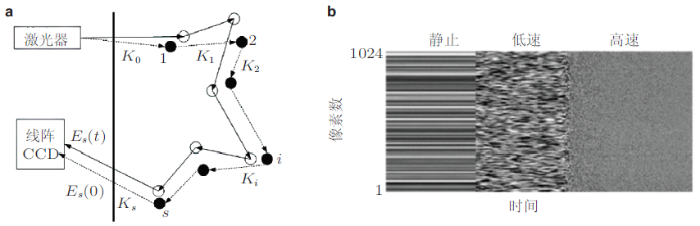SVS法最早由美国宾夕法尼亚大学Durian团队提出用于测量振动流化床表层颗粒的运动(Dixon & Durian 2002), 如图9所示在第一个1/4个周期, 玻璃珠随流化床一起以加速度$$1.25\sin \phi \cdot g$$上升, 玻璃珠间紧密挤压在一起, 颗粒温度几乎为0$$^{\circ}$$C; 在第二个1/4个周期, 流化床开始下降, 而玻璃珠由于惯性继续上升一段距离后开始下降, 在此过程中玻璃珠速度加快, 颗粒温度升高, 并在接近1/2周期时, 达到峰值(图9(b)中空心三角处); 在第三个1/4个周期, 随着玻璃珠掉落到流化床上并互相碰撞, 颗粒温度形成一个尖峰; 此后随着玻璃珠再次堆积在流化床上, 颗粒温度逐渐下降, 并在最后一个1/4个周期趋于0. 此后, Durian团队进一步对该技术进行了完善, 将其用于泡沫(foam)和胶体(colloidal suspension)等研究(Gittings & Durian 2008, Le et al. 2012, Ianni et al. 2006).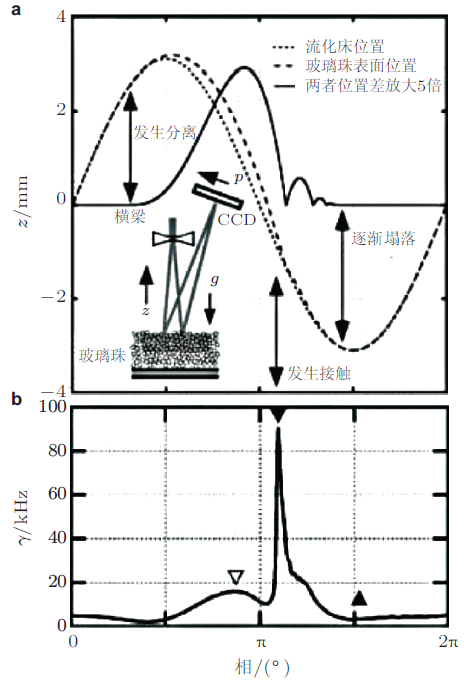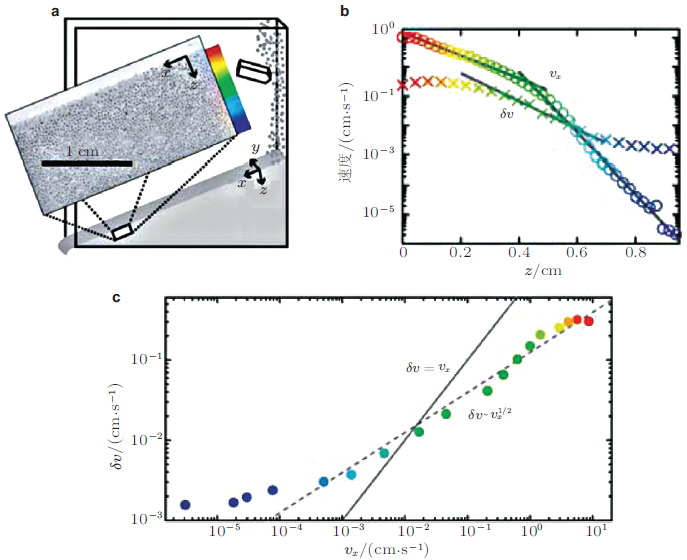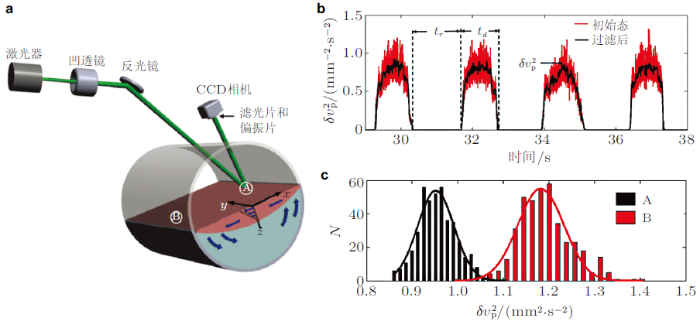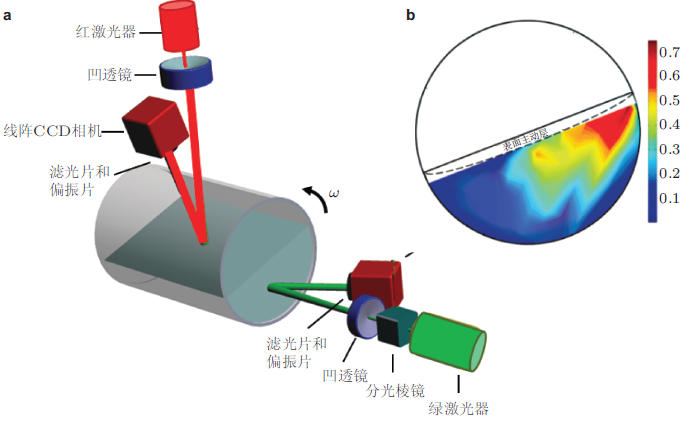SVS技术还可以和其他技术结合, 从不同角度对颗粒物质的动力学特性进行同步测量研究. 图13(a)所示为SVS技术与动态称重技术(测量颗粒物质崩塌过程中重力矩的变化)同步测量滚筒内不同黏性粉体的实验装置图(Yang et al.2016), 从图13(b)可以看到, 颗粒脉动速度(红色实线)与黑色虚线下降段所示谷底颗粒材料重力矩高度吻合, 此时颗粒相对静止.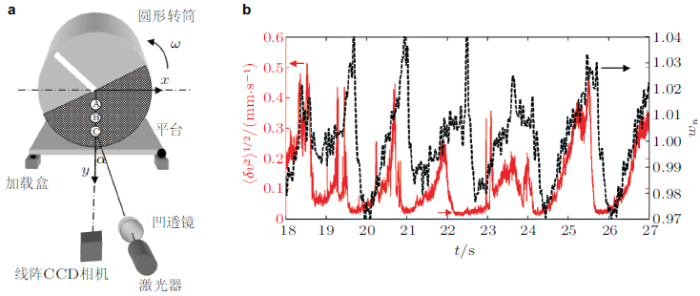## 4 透视测速法

### 4.1 正电子发射颗粒跟踪技术(PEPT)

PEPT是利用正电子成像(positron emission tomography, PET)技术, 通过追踪具有放射性标识的颗粒位置, 进而计算出颗粒流速度的技术.如图14所示其原理是利用同位素衰变时产生的正电子来实现显像的正电子发射型计算机断层扫描术, 具体为采用两个相对的探测器对正电子湮灭辐射产生的位于一条直线上、方向相反的两个光子产生的$$\gamma$$射线进行监测, 从而确定闪烁点位置.该系统包括一个正电子相机、跟踪剂位置和速度的定位算法以及跟踪标记技术.PEPT的示踪剂为发射正电子的放射性同位素, 其半衰期时间$$\left( {t_{1/ 2} } \right)$$应该保证实验完成,又要在使用后能够安全废弃, 通常使用的放射性同位素有: $${ }^{66}{\rm Ga}\left( {t_{1/2} =9.45\mbox{h}} \right)$$, $${ }^{68}{\rm Ga}\left( {t_{1/2} =68 {\rm min}} \right)$$, $${ }^{18}{\rm F}\left( {t_{1/2} =109 {\rm min}} \right)$$, $${ }^{61}{\rm Cu}\left( {t_{1/2} =2.7 {\rm h}} \right)$$和$${ }^{64}{\rm Cu}\left( {t_{1/2} =204 {\rm min}} \right)$$.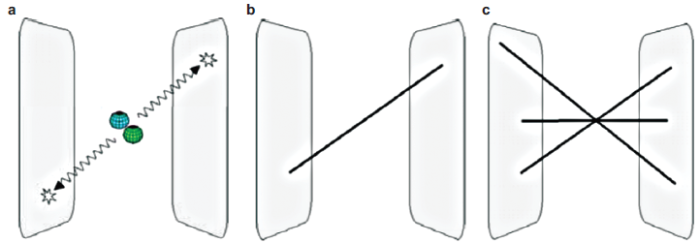PEPT技术最早由英国伯明翰大学的Parker课题组提出(Hawkesworth & Parker. 1991, Parker et al. 1993), 其追踪单个颗粒的空间分辨率可以达到0.5 mm, 时间分辨率主要由正电子相机决定（一般几十毫秒）, 最大可以测量10 m/s的颗粒速度, 最多可以追踪3个示踪颗粒.由于其可追踪的示踪颗粒数量较少, 实际应用中比较适合测量具有周期边界的循环实验装置, 如滚筒、鼓泡气体流化床、振动流化床等. 与其他非侵入式测量技术相比, PEPT技术能够测出更加详细的固/液运动信息, 并且能够测量液体、有机聚合物、金属和矿物颗粒等众多材料.其不足之处在于, PEPT能跟踪颗粒数较少, 不适合复杂颗粒流速度场分布的测量. 此外, PEPT给出的是关于时间的平均速度场信息, 而不是颗粒瞬时速度场信息, 因此只适用于测量稳定流. 图15所示为Parker 等(1997)用PEPT技术对准二维滚筒内(直径100 mm, 厚度16 mm, 颗粒直径3 mm)颗粒流速度的DEM计算结果进行了验证, 发现不同转速下, DEM计算的颗粒流表层速度总是大于PEPT的测量值, 造成这一结果主要是由于两方面原因, 一是DEM仿真时不考虑滚筒壁的作用, 二是DEM计算的速度是表层颗粒的平均速度, 而PEPT测得的是单个示踪颗粒的碰撞速度.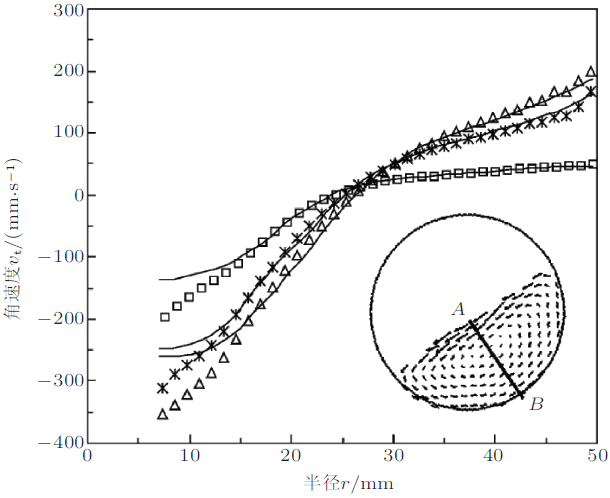Parker & Fan用PEPT技术测量了鼓泡流化床中的颗粒速度场分布(Parker & Fan 2008), 如图16(a)所示, 并根据速度场分布将流化床分为底部、中部和顶部三个区域. 图16(b)显示在流化床底部区域, 颗粒物质随着气泡在流化床边缘呈环状向上运动, 随后在流化床中间落下.这与图16(a)中顶部区域颗粒流的运动方向正好相反. 在中间区域, 图16(c)显示上升的气泡被从顶部边缘落下的颗粒挤到中间, 导致颗粒流速度场在流化床中间形成了一个小峰. 根据PEPT测量结果, 可以进一步计算出流化床中气泡的速度随高度变化, 如图16(d)所示.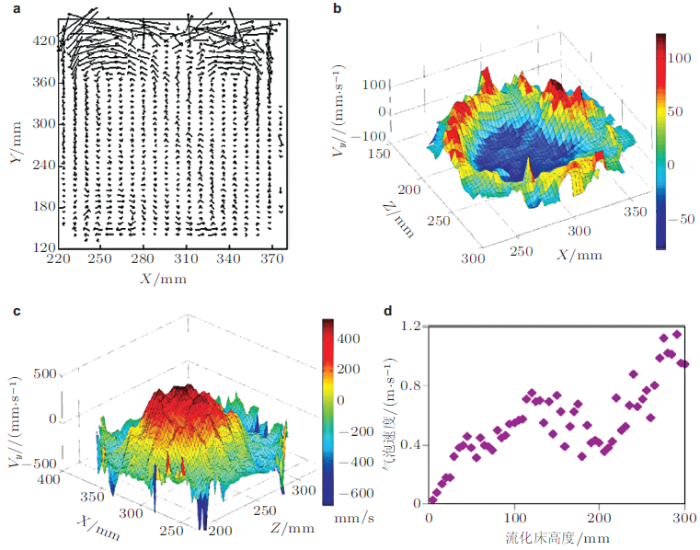### 4.2 磁共振测速技术(MRV)

MRV是利用核磁共振(nuclear magnetic resonance, NMR)和磁共振成像(magnetic resonance imaging, MRI)原理来测量空间体内的三维速度场的一种技术(Haacke et al. 1999, Sederman et al. 2007), 其工作介质通常为含氢原子的颗粒物质, 在外加磁场作用下, 原子核自旋系统逐步被磁化, 原子核以莫拉频率进动.在外部相同频率动射频脉冲激励下, 原子核产生磁共振现象, 而共振后恢复平衡状态的原子核会将吸收的射频脉冲能量释放出来, 探测器将这种磁共振信号转换成电信号再进行处理(如图17所示).由于原子核自旋的共振频率与外部所加磁场相关, 因此外部磁场通常存在较弱的剃度渐变, 依此来实现空间定位, 起到追踪测量颗粒运动的目的.选用的颗粒物质要么本身含有质子$$(^{1}$$H)比如芥菜籽、罂粟籽等; 要么是特殊制作包含质子的颗粒, 如胶囊中填充了液体维生素E(C$$_{29}$$H$$_{50}$$O$$_{2})$$等.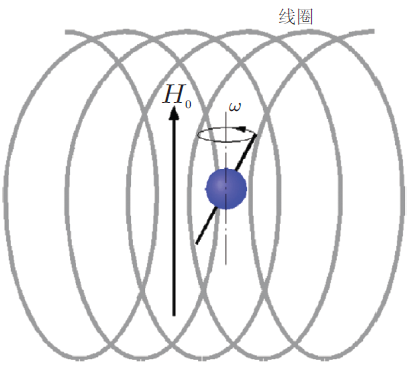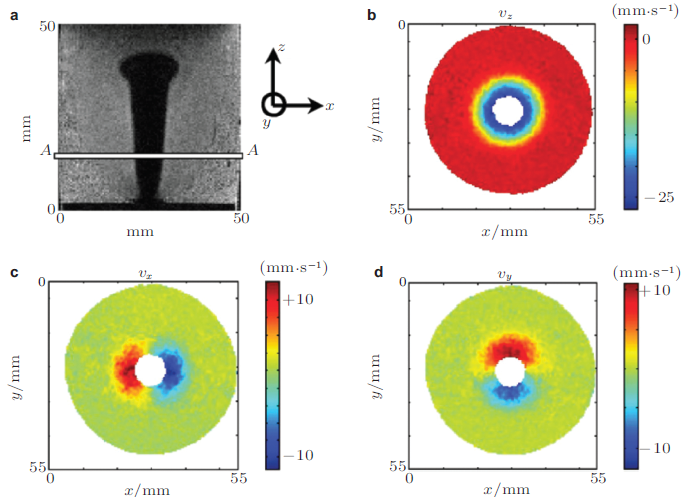### 4.3 同步辐射X射线成像技术(SR)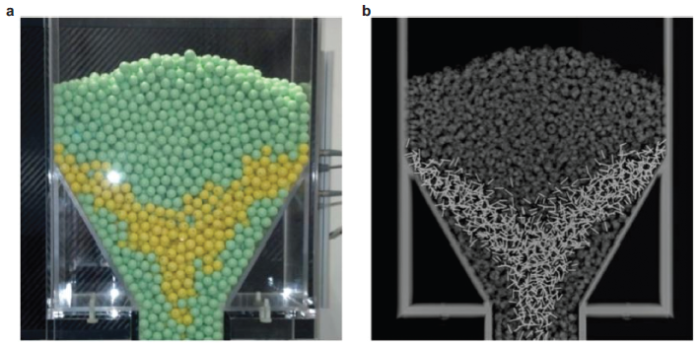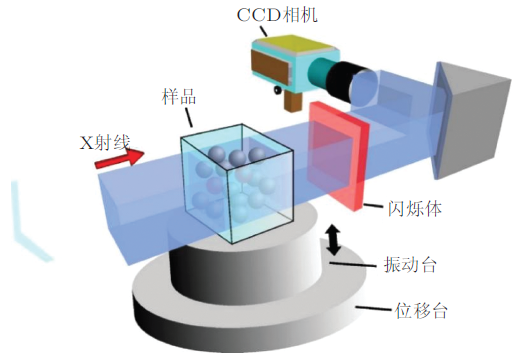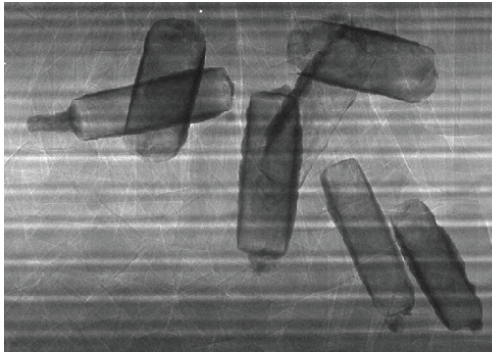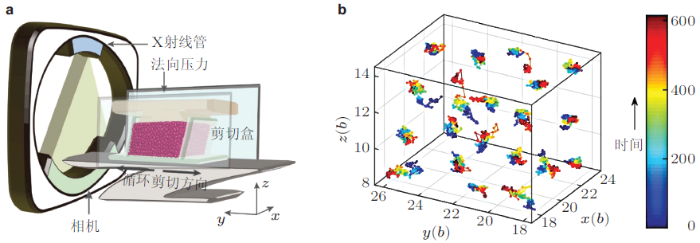## 5 介尺度结构的测量

### 5.1 二维力链结构的光弹技术(photoelastic technique)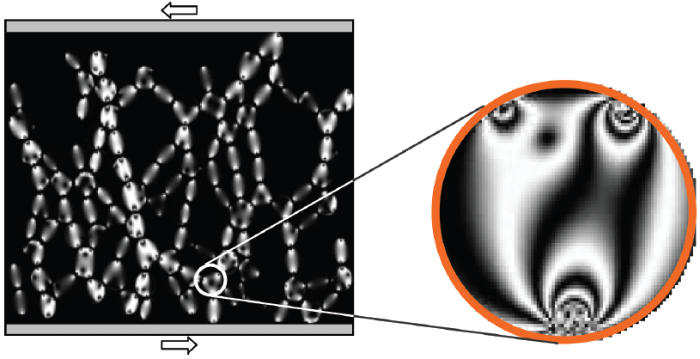### 5.2 声速测量技术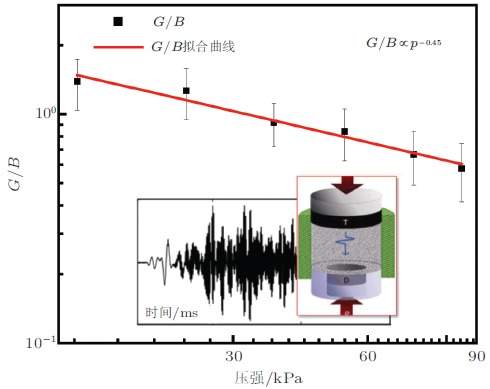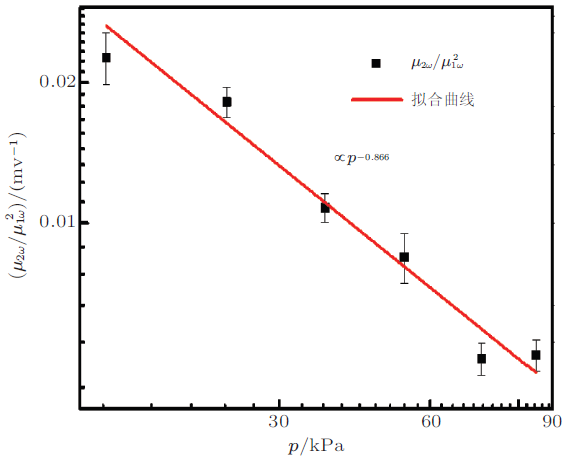### 5.3 体积谱技术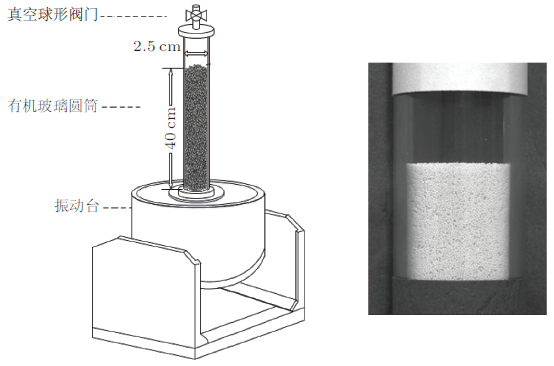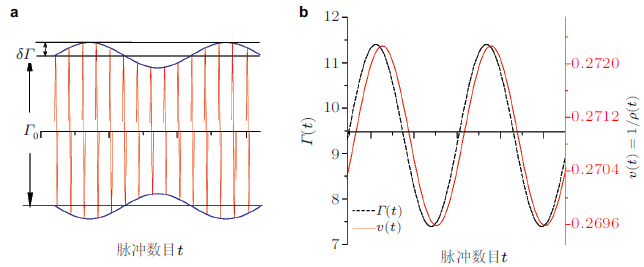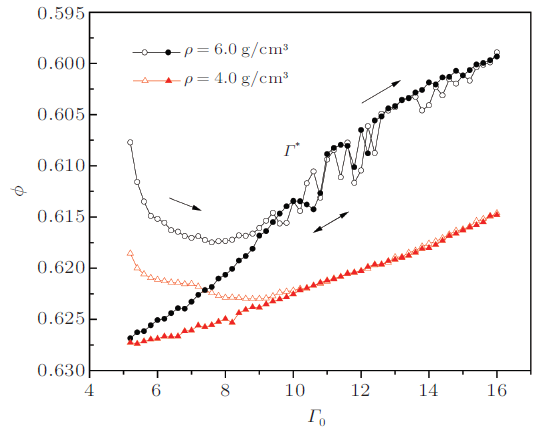### 5.4 垂直振动激励下颗粒材料有效质量和耗散功率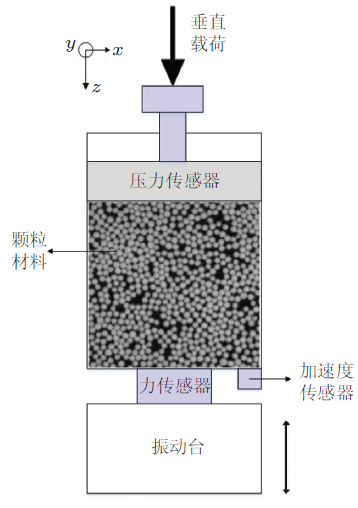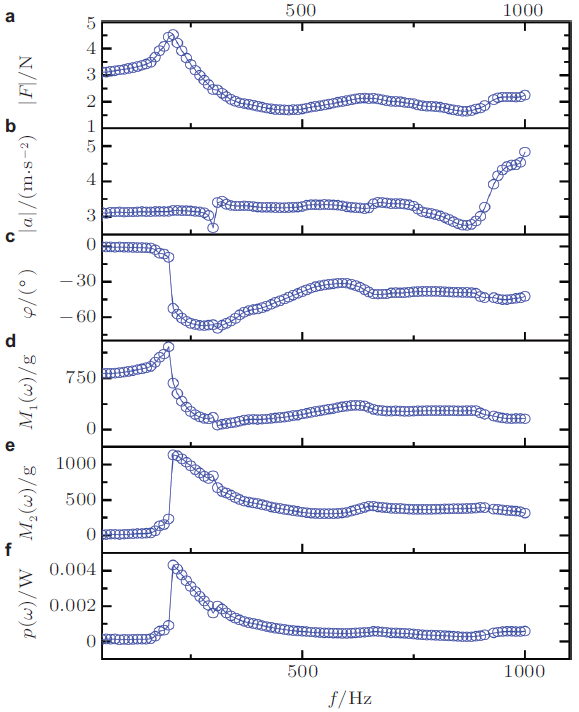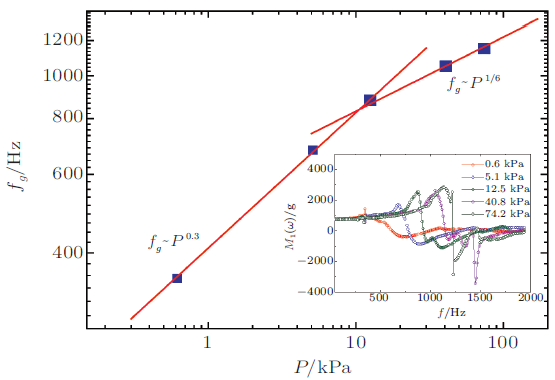$$Q$$值为共振频率与半峰高度的频宽的比值, 图34显示了颗粒系统品质因子倒数$$1 /Q$$随压强$$P$$的变化曲线, $$1/Q$$随$$P$$增大满足指数衰减规律, 即$\dfrac{1}{Q} = 0.4\exp \left( { - \dfrac{P}{4.25}} \right) + 0.08 \ \ (18)$这可能是由于表面压强较小时, 体系较为疏松, 颗粒内部结构更容易发生调整, 耗散效率较高. 增大颗粒上表面的压强, 实际上不仅增加了颗粒之间紧密和更均匀接触的数量, 而且减少了切线应力接触和潜在的滑动接触的数量, 因此$$1/ Q$$随压强的增大而减小. 当压强足够高时, 颗粒体系被压缩至接近固体的状态, $$1/ Q$$ 几乎不随压强变化.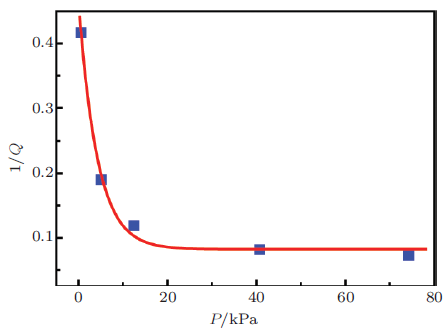## 6 结论与展望

(1)描述颗粒材料弹性、塑性和黏性的大量模型和理论需要实验测量结果评判其合理性和有效性, 必须加强发展测量颗粒结构和动力学的独特的实验技术, 为颗粒材料宏观性能研究提供科学依据. 比如, SVS技术、PEPT和SR技术等测得的是颗粒运动信息, 这些物理量与宏观塑性和黏性等基本性质相关; 内耗技术、DMA和声学谱等技术所测得的能量耗散和模量等都是宏观量, 这些物理量及其变化反映了颗粒材料内部发生的微观事件.比较可行的方法是, 针对颗粒材料结构特征, 对现有测量技术进行改造, 会遇到许多实际问题, 需要逐步予以完善使其适用.把这些测量技术在应用于颗粒体系时, 注意发现的新规律以及遇到的问题, 力图研发原创性的测量手段, 解决工程实践特定需求.由于颗粒体系内在过程复杂和多样, 建议开展多种技术联合测量, 多方面和多角度认识颗粒体系力学现象.

(2)实验测量到的是颗粒运动学量和结构量, 要建立其与宏观力学量的联系, 亦即需要发展颗粒材料的粗粒化方法.粗粒化方法确定微观(或介观的)的物理量与某一恰当的、较大尺度上刻画体系的物理量之间的联系(包括本构关系), 并建立其演化方程, 定性和定量分析它们对宏观力学现象的影响, 从介观结构层次全面解释颗粒体系力学性质.颗粒介质的粗粒化方法出现的较晚, Goldhirsh曾开展了理论推导, 由颗粒接触力得到应力$$\sigma _{ij}^\varepsilon$$, 以及由动量密度$$p_i$$得到应变$$\varepsilon _{ij}$$ 等等概念上还有不清晰的方面, 比如$$\sigma _{ij}^\varepsilon$$ 非对称性和偶应力量化, $$\varepsilon_{ij}$$定义中如何考虑颗粒自转等, 这些基本问题是颗粒介质所独有的.

(3)在透视测速领域, 目前的技术还不具有通用性, 这是由于一方面这些技术都需要极其专业而且昂贵的设备, 普通实验室很难实现; 另一方面, 这些技术都对人体健康有一定伤害, 如: PEPT, SR和CT等都具有辐射伤害, MRV虽然没有辐射但是长期在其强静磁场附近也会对人体产生影响. 因此, 开发一种安全、经济、具有普遍适用性的透视测速方法是当前迫切需要解决的问题.一种比较具有发展前景的技术就是采用无线传感技术的球形大颗粒运动测试技术, 该技术将各类传感器(如: 九轴运动传感器、温度传感器、应力传感器等)和2.4 G无线收发芯片, 通过单片机控制组成嵌入式系统构成测试节点, 植入颗粒内部(其中应变片贴在颗粒表面). 在颗粒运动过程中, 每个测试节点实时将测得的颗粒加速度和角速度、温度、以及应力等信息通过无线网络(局域网)发送到用户端, 用户端通过多线程智能采集技术, 高超采集各个节点的信息并计算出颗粒的速度、位移、角速度、应力等, 进而分析颗粒体系内部的速度流线、混流、停留时间、颗粒滑动和滚动等关键问题.但目前该技术的系统信噪比较差, 分辨率较低(空间分辨率: 1 cm以上, 时间分辨率: 0.1 s); 此外, 由于应力测量电路较复杂, 受体积的限制, 单个颗粒上应变片的数量有限(6个左右), 导致局部应力信息丢失. 因此, 选择精度更高、集成度更好的传感器, 以及开发适用性更强的信号处理算法(如卡尔曼滤波、深度学习等)是接下来重点研究方向.另一种安全的测试技术是目前最新的太赫兹(THz)技术, 太赫兹辐射是0.1 $$\sim$$ 10 THz的电磁辐射(介于无线电波中的毫米波和光波中的远红外线之间), 优点安全无辐射、具有较强的穿透性, 不足之处是成像精度低(空间分辨率: 5 cm以上), 扫描速度慢(由待测区域决定, 一般需要几分钟), 价格高, 目前国际上还没有采用该技术测量颗粒流相关报道.

(4)声学手段已经成为研究颗粒材料力学性质的重要手段.人们已经能够利用探测颗粒材料横纵波声速、剪切与体积模量比值$$G/K$$、声衰减系数等, 进而研究颗粒材料的塑性转变和声学衰减机制; 此外, 人们正在尝试利用非线性声学手段研究颗粒材料的非线性系数、弱力及缺陷分布等, 进而探测颗粒材料内部与散射相关的结构. 但是, 受到声源功率等的限制, 能够直接进行颗粒材料声学测量(特别是非线性声学测量)的商业化仪器还不多见, 还需要研发新的声学测量手段以满足工程实践的需求.

The authors have declared that no competing interests exist.

## 参考文献 原文顺序 文献年度倒序 文中引用次数倒序 被引期刊影响因子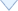〈〉